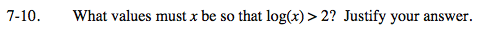Home > INT3 > Chapter 7 > Lesson 7.1.1 > Problem7-10

7-10.

What values must x be so that log(x) > 2? Justify your answer. Homework Help ✎Solve the equation log(x) = 2, and then test values to find where log(x) > 2.

Remember log(x) means log10(x). Rewrite the equation in exponential form.